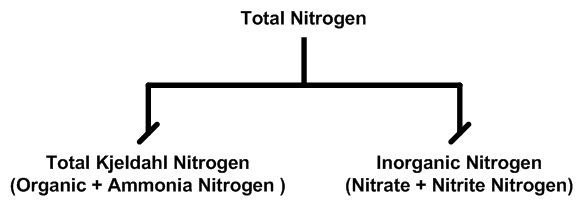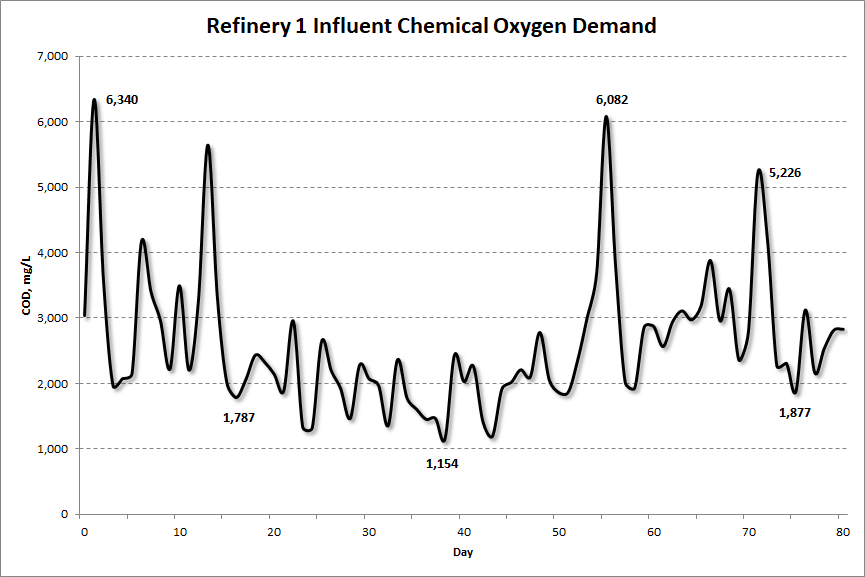# Nitrogen Testing

Updated: Nov 23, 2020

## Introduction

In a recent post I focused on the importance and value of total kjeldahl nitrogen (TKN) testing. That post addressed the need to run the full gamut of nitrogen tests which include TKN, ammonia, nitrate, and nitrite, the sum of which equate to the total nitrogen concentration as illustrated in Figure 1. In this post I want to focus on what is required to run these nitrogen tests by detailing the equipment and reagents I use in the field. Along the way I will give you a few tips.

Figure 1: Total Nitrogen Fractions## Total Kjeldahl Nitrogen (TKN) Testing

The TKN test is the most involved of the nitrogen tests. For all of my nitrogen testing I use Hach's TNT (test-in-a-tube) reagents. These reagent sets are by far the easiest to use but they do require sophisticated equipment (spectrophotometer and digital reactor) which I'll describe below. Hach's TKN test kit is shown in Figure 2. The image gives you an idea of the several steps required to run TKN. Though the steps are relatively many, this method is going to be the most user-friendly you will find.

Figure 2: Hach TKN TNT Reagent Set

For an overview of Hach's TKN test take a look at this PDF document. This is a one-page brochure.

To review the actual lab procedures for Hach's TKN test look at this PDF document.

## Sample Dilution Required!

A sometimes difficult task when doing testing at a new site is figuring out the range of concentrations you are working with. The TKN test measures the concentration in a range from 0 to 16 mg/L N. I have never tested an influent wastewater sample with a TKN concentration ≤16 mg/L (see Table 1). That means you are going to have to do a sample dilution. But what dilution? The TKN test requires heating your sample for one hour at 100°C. This is valuable time so you need to get your dilution right because you don't want to be over-range requiring that you rerun the test, losing an hour in the process.

Table 1: Metcalf & Eddy Nitrogen Concentrations## So What Dilution Should Be Used?

Since the TKN concentration is the sum of the organic nitrogen and the ammonia nitrogen I always run the ammonia nitrogen test first. Hach's TNT test for ammonia is a simple, quick, 15-minute test. Once I know the ammonia concentration I can get a feel for what the additional organic nitrogen contribution might be, depending on the type of wastewater plant I'm evaluating. But I can begin with the assumption that the TKN concentration is going to be approximately 40% greater than the ammonia concentration using Table 2 as a guide. Table 2 is derived from the M & E data shown in Table 1 above.

Table 2: Organic and Ammonia Nitrogen Percent DistributionBecause I'm often working in industrial facilities with high ammonia concentrations I start my testing by running two ammonia tests using two different ranges. Hach's Ammonia TNT test comes in three ranges, listed below, but I rarely use the ultra low range. Links are provided so you can download the lab procedures to see what is required to run each test.

1. Ultra Low Range (ULR), 0.015 to 2.00 mg/L NH3-N

2. High Range (HR), 2 to 47 mg/L NH3-N

3. Ultra High Range (UHR), 47 to 130 mg/L NH3-N

Figure 3: Hach High Range Ammonia TNT Test

Figure 4: Hach Ultra High Range Ammonia TNT Test

If I don't know what the ammonia concentration might be I'll run the HR ammonia (2 to 47 mg/L) and ULR (47 to 130 mg/L) knowing one of these tests will be outside the range the test procedure spans. If I am over range on the HR test, getting a concentration of 59 mg/L, for example, I can assume the TKN concentration will be approximately 40% greater than the ammonia concentration, based on Table 2 above, giving me a TKN value somewhere around 59 x 1.4 = 82.6 mg/L N. Since the upper limit of the TKN test is 16 mg/L N, my dilution needs to be ≥ (82.6 ÷ 16 = 5.16). In practical terms, this would result in doing a 1 to 6 dilution. A 1 to 6 dilution will give an upper limit of 6 x 16 = 96 mg/L N. I use an Excel spreadsheet, shown in Figure 5, to easily determine the resulting concentration and volumes required for the dilution.

Figure 5: Dilution Calculator, Ratio 1 to 6## Interference from COD

Here is another point you want to keep in mind, a point that will also influence your choice of a dilution ratio. Hach’s TKN TNT test will experience interference when the chemical oxygen demand (COD) of the sample is ≥ 500 mg/L. COD data is typically available so it is likely you won’t have to first run a two hour COD test before setting up for the hour long TKN test. But industrial wastewater plants can have highly variable influent COD concentrations so you need to make sure you have a good understanding of the influent organic loading. The graph in Figure 6 gives an example of just how variable the COD concentration can be. This is actual data from a refinery.

Figure 6: Highly Variable Influent COD ConcentrationsWith the variability shown in Figure 6, what assumptions can we make about the potential for COD to interfere with our TKN testing? The influent COD at this refinery is highly variable so we have to be conservative in deciding on the dilution ratio. By conservative I mean a higher ratio should be picked. In this case I'd probably go with a 1 to 11 ratio (5,226 ÷ 500 = 10.45). Otherwise I would have no choice but to first run a COD test before proceeding to measure the TKN concentration.

## Lab Equipment Required for Nitrogen Testing

For COD and TKN testing using Hach's TNT chemisty a spectrophotometer and a digital reactor are required. I use Hach's DR 1900 spectrophotometer pictured in Figure 7. This is a superb unit, rugged and relatively portable, which I ship all around the US as I go from one site to another.

Figure 7: Hach DR 1900 Spectrophotometer

Hach's digital reactor, required to heat samples, is shown in Figure 8. I recommend you get the dual bank unit shown in Figure 8. I also recommend you get the reactor configured to handle to size ranges for the vials as follows: 21x13mm vial wells, 4x20mm vial wells.

Figure 8: Hach Digigal Reactor

For all of Hach"s TNT testing you will be measuring small amounts of sample and reagents into 13-mm or 20-mm reaction vials. This calls for precision and the best way to achieve the necessary level of precision is to use a pipet. I use Hach's Tensette Pipets. These come in to ranges:

1. 0.1 to 1.0 mL and

2. 1.0 to 10.0 mL

I have both and you will want/need both as well. The larger volume pipet is shown in Figure 9.

Figure 9: Hach Tensette Pipet, 1.0 to 10.0 mL Volume

Finally, you will need a vial cooling rack so things aren't rolling around the lab bench and falling onto the floor. I use the rack shown in Figure 10.

Figure 10: Hach Stainless Steel Cooling Rack